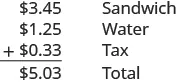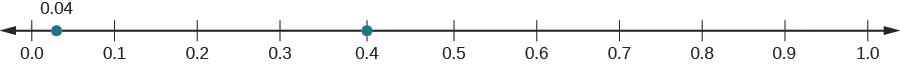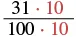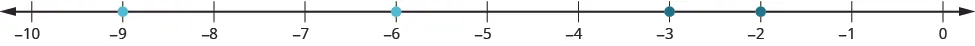Prealgebra

# 5.1Decimals

Prealgebra5.1 Decimals

### Learning Objectives

By the end of this section, you will be able to:
• Name decimals
• Write decimals
• Convert decimals to fractions or mixed numbers
• Locate decimals on the number line
• Order decimals
• Round decimals

### Be Prepared 5.1

Before you get started, take this readiness quiz.

1. Name the number $4,926,0154,926,015$ in words.
If you missed this problem, review Example 1.4.
2. Round $748748$ to the nearest ten.
If you missed this problem, review Example 1.9.
3. Locate $310310$ on a number line.
If you missed this problem, review Example 4.16.

### Name Decimals

You probably already know quite a bit about decimals based on your experience with money. Suppose you buy a sandwich and a bottle of water for lunch. If the sandwich costs $3.453.45$, the bottle of water costs $1.251.25$, and the total sales tax is $0.330.33$, what is the total cost of your lunch?The total is $5.03.5.03.$ Suppose you pay with a $55$ bill and $33$ pennies. Should you wait for change? No, $55$ and $33$ pennies is the same as $5.03.5.03.$

Because $100 pennies=1,100 pennies=1,$ each penny is worth $11001100$ of a dollar. We write the value of one penny as $0.01,0.01,$ since $0.01=1100.0.01=1100.$

Writing a number with a decimal is known as decimal notation. It is a way of showing parts of a whole when the whole is a power of ten. In other words, decimals are another way of writing fractions whose denominators are powers of ten. Just as the counting numbers are based on powers of ten, decimals are based on powers of ten. Table 5.1 shows the counting numbers.

Counting number Name
$11$ One
$10=1010=10$ Ten
$10·10=10010·10=100$ One hundred
$10·10·10=100010·10·10=1000$ One thousand
$10·10·10·10=10,00010·10·10·10=10,000$ Ten thousand
Table 5.1

How are decimals related to fractions? Table 5.2 shows the relation.

Decimal Fraction Name
$0.10.1$ $110110$ One tenth
$0.010.01$ $11001100$ One hundredth
$0.0010.001$ $11,00011,000$ One thousandth
$0.00010.0001$ $110,000110,000$ One ten-thousandth
Table 5.2

When we name a whole number, the name corresponds to the place value based on the powers of ten. In Whole Numbers, we learned to read $10,00010,000$ as ten thousand. Likewise, the names of the decimal places correspond to their fraction values. Notice how the place value names in Figure 5.2 relate to the names of the fractions from Table 5.2.

Figure 5.2 This chart illustrates place values to the left and right of the decimal point.

Notice two important facts shown in Figure 5.2.

• The “th” at the end of the name means the number is a fraction. “One thousand” is a number larger than one, but “one thousandth” is a number smaller than one.
• The tenths place is the first place to the right of the decimal, but the tens place is two places to the left of the decimal.

Remember that $5.035.03$ lunch? We read $5.035.03$ as five dollars and three cents. Naming decimals (those that don’t represent money) is done in a similar way. We read the number $5.035.03$ as five and three hundredths.

We sometimes need to translate a number written in decimal notation into words. As shown in Figure 5.3, we write the amount on a check in both words and numbers.

Figure 5.3 When we write a check, we write the amount as a decimal number as well as in words. The bank looks at the check to make sure both numbers match. This helps prevent errors.
 Let’s try naming a decimal, such as 15.68. We start by naming the number to the left of the decimal. fifteen______ We use the word “and” to indicate the decimal point. fifteen and_____ Then we name the number to the right of the decimal point as if it were a whole number. fifteen and sixty-eight_____ Last, name the decimal place of the last digit. fifteen and sixty-eight hundredths

The number $15.6815.68$ is read fifteen and sixty-eight hundredths.

### How To

#### Name a decimal number.

• Name the number to the left of the decimal point.
• Write “and” for the decimal point.
• Name the “number” part to the right of the decimal point as if it were a whole number.
• Name the decimal place of the last digit.

### Example 5.1

Name each decimal: $4.34.3$ $2.452.45$ $0.0090.009$ $−15.571.−15.571.$

### Try It 5.1

Name each decimal:

$6.76.7$ $19.5819.58$ $0.0180.018$ $−2.053−2.053$

### Try It 5.2

Name each decimal:

$5.85.8$ $3.573.57$ $0.0050.005$ $−13.461−13.461$

### Write Decimals

Now we will translate the name of a decimal number into decimal notation. We will reverse the procedure we just used.

Let’s start by writing the number six and seventeen hundredths:

 six and seventeen hundredths The word and tells us to place a decimal point. ___.___ The word before and is the whole number; write it to the left of the decimal point. 6._____ The decimal part is seventeen hundredths. Mark two places to the right of the decimal point for hundredths. 6._ _ Write the numerals for seventeen in the places marked. 6.17

### Example 5.2

Write fourteen and thirty-seven hundredths as a decimal.

### Try It 5.3

Write as a decimal: thirteen and sixty-eight hundredths.

### Try It 5.4

Write as a decimal: five and eight hundred ninety-four thousandths.

### How To

#### Write a decimal number from its name.

1. Step 1. Look for the word “and”—it locates the decimal point.
2. Step 2.
Mark the number of decimal places needed to the right of the decimal point by noting the place value indicated by the last word.
• Place a decimal point under the word “and.” Translate the words before “and” into the whole number and place it to the left of the decimal point.
• If there is no “and,” write a “0” with a decimal point to its right.
3. Step 3. Translate the words after “and” into the number to the right of the decimal point. Write the number in the spaces—putting the final digit in the last place.
4. Step 4. Fill in zeros for place holders as needed.

The second bullet in Step 2 is needed for decimals that have no whole number part, like ‘nine thousandths’. We recognize them by the words that indicate the place value after the decimal – such as ‘tenths’ or ‘hundredths.’ Since there is no whole number, there is no ‘and.’ We start by placing a zero to the left of the decimal and continue by filling in the numbers to the right, as we did above.

### Example 5.3

Write twenty-four thousandths as a decimal.

### Try It 5.5

Write as a decimal: fifty-eight thousandths.

### Try It 5.6

Write as a decimal: sixty-seven thousandths.

Before we move on to our next objective, think about money again. We know that $11$ is the same as $1.00.1.00.$ The way we write $1(or1.00)1(or1.00)$ depends on the context. In the same way, integers can be written as decimals with as many zeros as needed to the right of the decimal.

$5=5.0−2=−2.05=5.00−2=−2.005=5.000−2=−2.0005=5.0−2=−2.05=5.00−2=−2.005=5.000−2=−2.000$
$and so on…and so on…$

### Convert Decimals to Fractions or Mixed Numbers

We often need to rewrite decimals as fractions or mixed numbers. Let’s go back to our lunch order to see how we can convert decimal numbers to fractions. We know that $5.035.03$ means $55$ dollars and $33$ cents. Since there are $100100$ cents in one dollar, $33$ cents means $31003100$ of a dollar, so $0.03=3100.0.03=3100.$

We convert decimals to fractions by identifying the place value of the farthest right digit. In the decimal $0.03,0.03,$ the $33$ is in the hundredths place, so $100100$ is the denominator of the fraction equivalent to $0.03.0.03.$

$0.03=31000.03=3100$

For our $5.035.03$ lunch, we can write the decimal $5.035.03$ as a mixed number.

$5.03=531005.03=53100$

Notice that when the number to the left of the decimal is zero, we get a proper fraction. When the number to the left of the decimal is not zero, we get a mixed number.

### How To

#### Convert a decimal number to a fraction or mixed number.

1. Step 1.
Look at the number to the left of the decimal.
• If it is zero, the decimal converts to a proper fraction.
• If it is not zero, the decimal converts to a mixed number.
• Write the whole number.
2. Step 2. Determine the place value of the final digit.
3. Step 3.
Write the fraction.
• numerator—the ‘numbers’ to the right of the decimal point
• denominator—the place value corresponding to the final digit
4. Step 4. Simplify the fraction, if possible.

### Example 5.4

Write each of the following decimal numbers as a fraction or a mixed number:

$4.094.09$ $3.73.7$ $−0.286−0.286$

### Try It 5.7

Write as a fraction or mixed number. Simplify the answer if possible.

$5.35.3$ $6.076.07$ $−0.234−0.234$

### Try It 5.8

Write as a fraction or mixed number. Simplify the answer if possible.

$8.78.7$ $1.031.03$ $−0.024−0.024$

### Locate Decimals on the Number Line

Since decimals are forms of fractions, locating decimals on the number line is similar to locating fractions on the number line.

### Example 5.5

Locate $0.40.4$ on a number line.

### Try It 5.9

Locate $0.60.6$ on a number line.

### Try It 5.10

Locate $0.90.9$ on a number line.

### Example 5.6

Locate $−0.74−0.74$ on a number line.

### Try It 5.11

Locate $−0.63−0.63$ on a number line.

### Try It 5.12

Locate $−0.25−0.25$ on a number line.

### Order Decimals

Which is larger, $0.040.04$ or $0.40?0.40?$

If you think of this as money, you know that $0.400.40$ (forty cents) is greater than $0.040.04$ (four cents). So,

$0.40>0.040.40>0.04$

In previous chapters, we used the number line to order numbers.

$ab‘ais greater thanb’whenais to the right ofbon the number lineab‘ais greater thanb’whenais to the right ofbon the number line$

Where are $0.040.04$ and $0.400.40$ located on the number line?We see that $0.400.40$ is to the right of $0.04.0.04.$ So we know $0.40>0.04.0.40>0.04.$

How does $0.310.31$ compare to $0.308?0.308?$ This doesn’t translate into money to make the comparison easy. But if we convert $0.310.31$ and $0.3080.308$ to fractions, we can tell which is larger.

 $0.310.31$ $0.3080.308$ Convert to fractions. $3110031100$ $30810003081000$ We need a common denominator to compare them.$3081000 3081000$ $31010003101000$ $30810003081000$

Because $310>308,310>308,$ we know that $3101000>3081000.3101000>3081000.$ Therefore, $0.31>0.308.0.31>0.308.$

Notice what we did in converting $0.310.31$ to a fraction—we started with the fraction $3110031100$ and ended with the equivalent fraction $3101000.3101000.$ Converting $31010003101000$ back to a decimal gives $0.310.0.310.$ So $0.310.31$ is equivalent to $0.310.0.310.$ Writing zeros at the end of a decimal does not change its value.

$31100=3101000and0.31=0.31031100=3101000and0.31=0.310$

If two decimals have the same value, they are said to be equivalent decimals.

$0.31=0.3100.31=0.310$

We say $0.310.31$ and $0.3100.310$ are equivalent decimals.

### Equivalent Decimals

Two decimals are equivalent decimals if they convert to equivalent fractions.

Remember, writing zeros at the end of a decimal does not change its value.

### How To

#### Order decimals.

1. Step 1. Check to see if both numbers have the same number of decimal places. If not, write zeros at the end of the one with fewer digits to make them match.
2. Step 2. Compare the numbers to the right of the decimal point as if they were whole numbers.
3. Step 3. Order the numbers using the appropriate inequality sign.

### Example 5.7

Order the following decimals using $::$

1. $0.64__0.60.64__0.6$
2. $0.83__0.8030.83__0.803$

### Try It 5.13

Order each of the following pairs of numbers, using $::$

$0.42__0.40.42__0.4$ $0.76__0.7060.76__0.706$

### Try It 5.14

Order each of the following pairs of numbers, using $::$

$0.1__0.180.1__0.18$ $0.305__0.350.305__0.35$

When we order negative decimals, it is important to remember how to order negative integers. Recall that larger numbers are to the right on the number line. For example, because $−2−2$ lies to the right of $−3−3$ on the number line, we know that $−2>−3.−2>−3.$ Similarly, smaller numbers lie to the left on the number line. For example, because $−9−9$ lies to the left of $−6−6$ on the number line, we know that $−9<−6.−9<−6.$If we zoomed in on the interval between $00$ and $−1,−1,$ we would see in the same way that $−0.2>−0.3and−0.9<−0.6.−0.2>−0.3and−0.9<−0.6.$

### Example 5.8

Use $$ to order. $−0.1__−0.8.−0.1__−0.8.$

### Try It 5.15

Order each of the following pairs of numbers, using $::$

$−0.3___−0.5−0.3___−0.5$

### Try It 5.16

Order each of the following pairs of numbers, using $::$

$−0.6___−0.7−0.6___−0.7$

### Round Decimals

In the United States, gasoline prices are usually written with the decimal part as thousandths of a dollar. For example, a gas station might post the price of unleaded gas at $3.2793.279$ per gallon. But if you were to buy exactly one gallon of gas at this price, you would pay $3.283.28$, because the final price would be rounded to the nearest cent. In Whole Numbers, we saw that we round numbers to get an approximate value when the exact value is not needed. Suppose we wanted to round $2.722.72$ to the nearest dollar. Is it closer to $22$ or to $3?3?$ What if we wanted to round $2.722.72$ to the nearest ten cents; is it closer to $2.702.70$ or to $2.80?2.80?$ The number lines in Figure 5.4 can help us answer those questions.

Figure 5.4 We see that $2.722.72$ is closer to $33$ than to $2.2.$ So, $2.722.72$ rounded to the nearest whole number is $3.3.$
We see that $2.722.72$ is closer to $2.702.70$ than $2.80.2.80.$ So we say that $2.722.72$ rounded to the nearest tenth is $2.7.2.7.$

Can we round decimals without number lines? Yes! We use a method based on the one we used to round whole numbers.

### How To

#### Round a decimal.

1. Step 1. Locate the given place value and mark it with an arrow.
2. Step 2. Underline the digit to the right of the given place value.
3. Step 3.
Is this digit greater than or equal to $5?5?$
• Yes - add $11$ to the digit in the given place value.
• No - do not change the digit in the given place value
4. Step 4. Rewrite the number, removing all digits to the right of the given place value.

### Example 5.9

Round $18.37918.379$ to the nearest hundredth.

### Try It 5.17

Round to the nearest hundredth: $1.047.1.047.$

### Try It 5.18

Round to the nearest hundredth: $9.173.9.173.$

### Example 5.10

Round $18.37918.379$ to the nearest tenth whole number.

### Try It 5.19

Round $6.5826.582$ to the nearest hundredth tenth whole number.

### Try It 5.20

Round $15.217515.2175$ to the nearest thousandth hundredth tenth.

### Section 5.1 Exercises

#### Practice Makes Perfect

Name Decimals

In the following exercises, name each decimal.

1.

$5.5 5.5$

2.

$7.8 7.8$

3.

$5.01 5.01$

4.

$14.02 14.02$

5.

$8.71 8.71$

6.

$2.64 2.64$

7.

$0.002 0.002$

8.

$0.005 0.005$

9.

$0.381 0.381$

10.

$0.479 0.479$

11.

$−17.9 −17.9$

12.

$−31.4 −31.4$

Write Decimals

In the following exercises, translate the name into a decimal number.

13.

Eight and three hundredths

14.

Nine and seven hundredths

15.

Twenty-nine and eighty-one hundredths

16.

Sixty-one and seventy-four hundredths

17.

Seven tenths

18.

Six tenths

19.

One thousandth

20.

Nine thousandths

21.

Twenty-nine thousandths

22.

Thirty-five thousandths

23.

Negative eleven and nine ten-thousandths

24.

Negative fifty-nine and two ten-thousandths

25.

Thirteen and three hundred ninety-five ten thousandths

26.

Thirty and two hundred seventy-nine thousandths

Convert Decimals to Fractions or Mixed Numbers

In the following exercises, convert each decimal to a fraction or mixed number.

27.

$1.99 1.99$

28.

$5.83 5.83$

29.

$15.7 15.7$

30.

$18.1 18.1$

31.

$0.239 0.239$

32.

$0.373 0.373$

33.

$0.13 0.13$

34.

$0.19 0.19$

35.

$0.011 0.011$

36.

$0.049 0.049$

37.

$−0.00007 −0.00007$

38.

$−0.00003 −0.00003$

39.

$6.4 6.4$

40.

$5.2 5.2$

41.

$7.05 7.05$

42.

$9.04 9.04$

43.

$4.006 4.006$

44.

$2.008 2.008$

45.

$10.25 10.25$

46.

$12.75 12.75$

47.

$1.324 1.324$

48.

$2.482 2.482$

49.

$14.125 14.125$

50.

$20.375 20.375$

Locate Decimals on the Number Line

In the following exercises, locate each number on a number line.

51.

$0.8 0.8$

52.

$0.3 0.3$

53.

$−0.2 −0.2$

54.

$−0.9 −0.9$

55.

$3.1 3.1$

56.

$2.7 2.7$

57.

$−2.5 −2.5$

58.

$−1.6 −1.6$

Order Decimals

In the following exercises, order each of the following pairs of numbers, using $..$

59.

$0.9 __ 0.6 0.9 __ 0.6$

60.

$0.7 __ 0.8 0.7 __ 0.8$

61.

$0.37 __ 0.63 0.37 __ 0.63$

62.

$0.86 __ 0.69 0.86 __ 0.69$

63.

$0.6 __ 0.59 0.6 __ 0.59$

64.

$0.27 __ 0.3 0.27 __ 0.3$

65.

$0.91 __ 0.901 0.91 __ 0.901$

66.

$0.415 __ 0.41 0.415 __ 0.41$

67.

$−0.5 __ −0.3 −0.5 __ −0.3$

68.

$−0.1 _ −0.4 −0.1 _ −0.4$

69.

$−0.62 _ −0.619 −0.62 _ −0.619$

70.

$−7.31 _ −7.3 −7.31 _ −7.3$

Round Decimals

In the following exercises, round each number to the nearest tenth.

71.

$0.67 0.67$

72.

$0.49 0.49$

73.

$2.84 2.84$

74.

$4.63 4.63$

In the following exercises, round each number to the nearest hundredth.

75.

$0.845 0.845$

76.

$0.761 0.761$

77.

$5.7932 5.7932$

78.

$3.6284 3.6284$

79.

$0.299 0.299$

80.

$0.697 0.697$

81.

$4.098 4.098$

82.

$7.096 7.096$

In the following exercises, round each number to the nearest hundredth tenth whole number.

83.

$5.781 5.781$

84.

$1.638 1.638$

85.

$63.479 63.479$

86.

$84.281 84.281$

#### Everyday Math

87.

Salary Increase Danny got a raise and now makes $58,965.9558,965.95$ a year. Round this number to the nearest:

dollar

thousand dollars

ten thousand dollars.

88.

New Car Purchase Selena’s new car cost $23,795.95.23,795.95.$ Round this number to the nearest:

dollar

thousand dollars

ten thousand dollars.

89.

Sales Tax Hyo Jin lives in San Diego. She bought a refrigerator for $1624.991624.99$ and when the clerk calculated the sales tax it came out to exactly $142.186625.142.186625.$ Round the sales tax to the nearest penny dollar.

90.

Sales Tax Jennifer bought a $1,038.991,038.99$ dining room set for her home in Cincinnati. She calculated the sales tax to be exactly $67.53435.67.53435.$ Round the sales tax to the nearest penny dollar.

#### Writing Exercises

91.

92.

Explain how you write “three and nine hundredths” as a decimal.

93.

Jim ran a $100-meter100-meter$ race in $12.32 seconds.12.32 seconds.$ Tim ran the same race in $12.3 seconds.12.3 seconds.$ Who had the faster time, Jim or Tim? How do you know?

94.

Gerry saw a sign advertising postcards marked for sale at $“10for0.99¢.”“10for0.99¢.”$ What is wrong with the advertised price?

#### Self Check

After completing the exercises, use this checklist to evaluate your mastery of the objectives of this section.

If most of your checks were:

…confidently. Congratulations! You have achieved the objectives in this section. Reflect on the study skills you used so that you can continue to use them. What did you do to become confident of your ability to do these things? Be specific.

…with some help. This must be addressed quickly because topics you do not master become potholes in your road to success. In math, every topic builds upon previous work. It is important to make sure you have a strong foundation before you move on. Who can you ask for help? Your fellow classmates and instructor are good resources. Is there a place on campus where math tutors are available? Can your study skills be improved?

…no—I don’t get it! This is a warning sign and you must not ignore it. You should get help right away or you will quickly be overwhelmed. See your instructor as soon as you can to discuss your situation. Together you can come up with a plan to get you the help you need.

Order a print copy

As an Amazon Associate we earn from qualifying purchases.